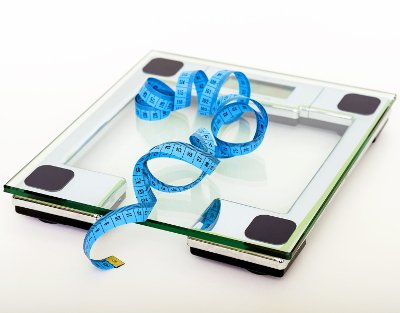# YMCA Body Fat Percentage Calculator

Share:

*
*
lb
Pounds
kg
Kilograms
*
in
Inches
cm
Centimeters

Similar Fitness Calculators

## About YMCA Body Fat Percentage CalculatorThis calculator is used to calculate the body fat percentage based on formula developed by YMCA.

### Using YMCA Body Fat Percentage calculator

1. Select the Gender.
2. Enter the Weight either in pounds (lb.) or in kilogram (kg).
3. Enter the Waist circumference either in inches (in) or centimeters (cm). (See guidelines below for taking measurement.)
4. Finally, click the Calculate YMCA %BF button. The body fat percentage based on YMCS formula will be calculated and the result will be displayed in the box below the button.

#### Guide For Measuring Waist Circumference

1. Use a self-tensioning taping device either digital or non-digital.
2. Wear little cloth as possible.
3. Stand straight with your feet close together.
4. Keep your arms at the sides.
5. Take the measurement after the end of a normal expiration.
6. Measure the circumference of the waist at the belly button (or navel). Repeat the measurements twice and take the average.

## YMCA Body Fat Percentage

This percent body fat calculation was devised by YMCA. This method uses sex, body weight and waist circumference to calculate the body fat percentage. There are separate formulas for men and women. Here are the formulas.

### Formula

There are different formulas for men and women.

#### YMCA Formula for Men

((4.15 × waist - 0.082 × weight - 98.42) / weight) × 100

Where:

• weight = Body weight in pounds.
• waist = Waist circumference in inches.

#### BFP Formula for Women

((4.15 × waist - 0.082 × weight - 76.76) / weight) × 100

Where:

• weight = Body weight in pounds.
• waist = Waist circumference in inches.

### Fat % Fitness Category

BFP Category Women Men
Essential Fat Percent10 - 13%2 - 5%
Fat Percent for Athletes14 - 20%6 - 13%
Fitness Level21 - 24%14 - 17%
Average Level25 - 31%18 - 24%
Obese Level32% and above25% and above